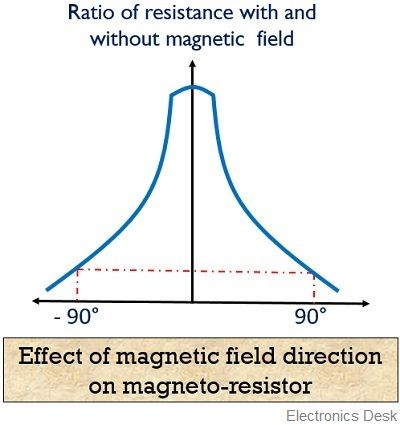# Magneto Resistors

Definition: Magneto resistors are those components that exhibit the property of magnetoresistance. Basically, magnetoresistance is the phenomenon of change in resistance of a material when a magnetic field is applied. The current that flows through a magneto resistor, varies with the variation in the applied magnetic field.

The resistivity of magnetoresistor depends on the mobility of electrons. Semiconductor materials like indium antimonide or indium arsenide are used for fabrication of magneto resistors.

Usually, the effect of magnetic field on the resistance of a material depends on the strength as well as the orientation of the magnetic field wrt current.

It is noteworthy here that, the sensitivity of magneto resistors depends on the total applied magnetic field and do not rely on the rate of change of the magnetic field.

The figure below shows the symbolic representation of magnetoresistor:## Construction of Magneto resistors

A magnetoresistor is basically formed by the deposition of a film of indium antimonide or nickel antimonide over a substrate of 0.1 m thickness. The thickness of the depositing film is about 25 µm.

In order to have variation in the resistance of the material in the absence of a magnetic field, the dimensions of the indium film are changed.

A plastic or ceramic material forms the insulation between substrate and indium film. These are basically non-magnetic material which is coated with a magnetic base.

## Working principle of Magneto resistors

A magnetoresistor operates on the law of electrodynamics. According to this law, in the presence of a magnetic field, Lorentz force is experienced by charge carriers. Hence, the electrons move in a different manner thereby causing the resistivity of increase with a decrease in current.

With the increase in strength of the magnetic field, resistance also increases and vice-versa.

When an external magnetic field is not applied, then the mobile charge carriers present inside the material follows a direct straight path. Due to this, electric current also flows in a straight path thus resistance, in that case, will be low.

As against, when a magnetic field is applied externally, then due to Lorentz force the electrons instead of flowing through direct path now starts following an indirect path. This causes the resistance of the material to increase.

The value of magnetoresistance depends on the magnetic field direction. The figure below shows the effect of the magnetic field direction on magneto resistors:It is to be noted here that when the crystals of indium antimonide are parallelly placed wrt each other. Also, the magnetic and electric field are perpendicular to each other, then maximal resistance change in the material occurs.

In some metals, like bismuth magnetoresistive effect can be seen at room temperature also. However, when the external magnetic field of 1.5 Χ 106 A/m is applied to bismuth, then its resistivity becomes twice of the resistivity in the absence of magnetic field. Hence, it can be used in the measurement of the flux density.

### Characteristic curve of Magneto resistor

The figure here represents the basic magneto-resistive characteristics:Here, H0 represents the effective anisotropy field in the material. When no external field is applied then the magnetization of the field exists at elemental length 0. But, with the increase in the applied field, the resistance also increases, this is represented by point a, in the above curve.

With further increase in the applied field saturation is reached that is shown by point b.

### Type of Magnetoresistive Effect

• Ordinary magnetoresistance: It is abbreviated as OMR and is basically a result of cyclic motion of electrons in the magnetic field. When the applied magnetic field is low, then resistance is somewhat low. However, with the rise in the applied magnetic field, the resistance of the material also increases.

For all the metals, the change in resistance of the metal is positive wrt applied magnetic field.

• Giant magnetoresistance: It is basically an effect which is noticed in multiple thin film alternating layers of metals and non-metals. Here, in this case, the change in resistance of the material is associated with the magnetization of the ferromagnetic layers in either parallel or antiparallel arrangement.

In GMR, when the magnetization of the ferromagnetic layers is parallel, then the resistance of the material is low. But in case of the antiparallel orientation of magnetic layers, the resistance comparatively increases.

• Anisotropic magnetoresistance: It is abbreviated as AMR. Here the resistance of the device depends on the angle between the direction of current and the arrangement of the magnetic field.

Basically, the rotational change in direction of magnetization somewhat distorts electron cloud. This deformation causes variation in the scattering of the conducting electrons.

• Tunneling magnetoresistance: It is sometimes abbreviated as TMR. Here, an insulator is present between 2 ferromagnetic layers. In case of small barrier applied by the insulator, electrons undergo easy tunneling from one ferromagnetic layer to the other thereby generating current. But, when this barrier is high, the current through the material decreases.

So, the tunneling current in these devices is high when the two ferromagnetic layers are aligned parallelly with each other as compared to antiparallel orientation.

### Applications of magneto resistors

These are widely used in magnetometers in order to determine the direction and intensity of magnetic field. Also, these are used in position sensors and ferrous metal detection.